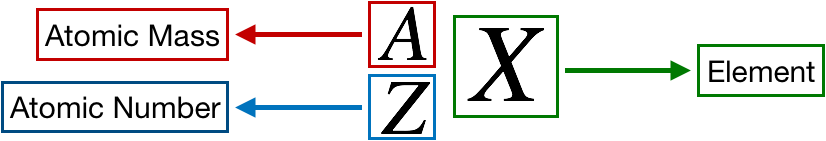# Problem: Fill in the gaps in the following table:SymbolCo3+Protons347680Neutrons46116120Electrons3678Mass number59Net charge2+Complete the first row of the table.

###### FREE Expert Solution

We’re being asked to complete the first row of the table.

Recall that the symbol for an element or ion is as follows:Recall that:

• Atomic Mass = A number of protons + number of neutrons
• Atomic NumberZ = number of protons = number of electrons
• Net charge
• number of electrons lost if positive (+)
• number of electrons gained if negative (-)

The number of protons for each element is given in the second row.

For the 2nd Column

• Recall that the number of protons is equal to the atomic number, thus in the periodic table, it is element 34 which is Selenium (Se).
84% (299 ratings)###### Problem Details
Fill in the gaps in the following table:

 Symbol Co3+ Protons 34 76 80 Neutrons 46 116 120 Electrons 36 78 Mass number 59 Net charge 2+

Complete the first row of the table.